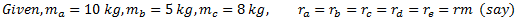## Friday, 3 March 2017

### Answer.Graphical Method: Component Mass(m) (kg) Radius(r)(m) mr(kgm) A 10 r 10r B 5 r 5r C 8 r 8r D E  mdr mcr r r  mcr mcr

From the tabular data and statement of the problem, it is clear that the system is in complete balance. Therefore, the force polygon should be a closed one. Hence it is drawn with the help of data in column 4 of the table as under:
2. From point a , draw line ab parallel to OB and equal to 5r.
3. Similarly, draw line bc parallel to OC and equal to 8r.
4 From point c, draw line cd parallel to OD.
5. From point O, draw line ed parallel to OE to cut line cd at point d.
6. Measure cd and equate it to mdr to find the value of md.
7. Similarly, measure de and equate it to mer . Find the value of me .

Let us now solve the above problem by analytical method.
Analytical Method: {see fig. a}
 Component Mass (m)(kg) Radius (r) (m) mr (kg m) mr sin θ A 0 r 10r 10r 0 B 5 r 5r 2.5r 4.33 C 8 r 8r -5.657r 5.657r D md r mdr -0.8666mdr -0.5 E me r mer 0 -mer

For complete balance,

From equation (i),

From equation (ii),